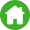Home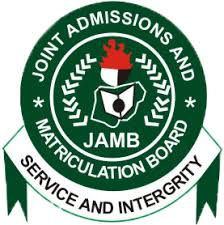Jamb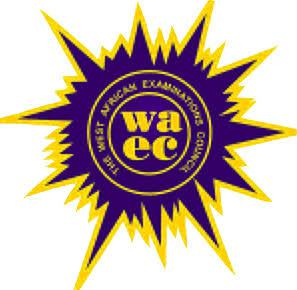Waec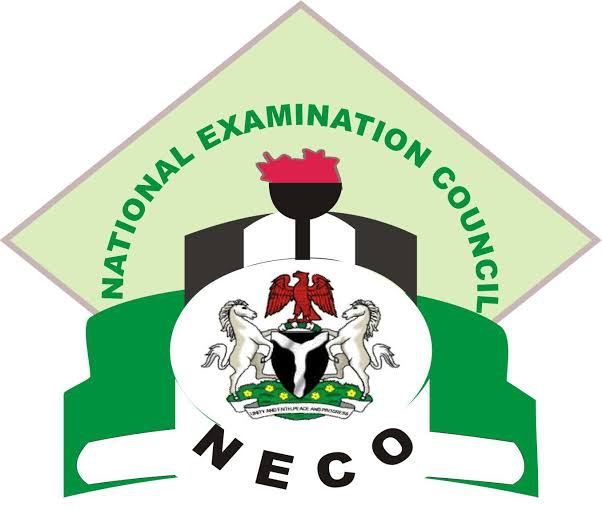Neco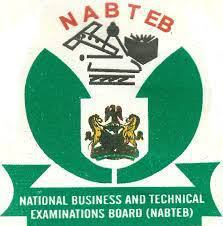NabtebNews

### SUBSCRIBE HERE!!

Quick Contact08162563540

--NECO 2019 TIMETABLE
--NECO 2019 SPECIMENS/PRACTICALSCLICK HERE FOR OUR NEW ANSWER LINK

# WAEC 2019/2020 General Mathematics Syllabus Questions and Answers

It is no longer news that Waec 2019/2020 registration has begun and the May/June examination is very close. So many waec candidates have been asking questions about 2019 waec syllabus and topics to read so as to pass waec 2019 without much stress.

The truth of the matter is that, the relevance of Jamb syllabus and expo on the topics to focus on cannot be overemphasized. There are four weapons you need you need to pass the WAEC 2019/2020 examination. They are:

WAEC Syllabus
Hot topics to read to pass waec 2019/2020
The recommended waec textbooks and

WAEC MATHEMATICS GENERAL GUIDE
For all papers which involve mathematical calculations, mathematical and statistical tables published for WAEC should be used in the examination room. However, the use of non-programmable, silent and cordless calculator is allowed.
The calculator must not have a paper printout. Where the degree of accuracy is not specified in a question the degree of accuracy expected will be that obtainable from the WAEC mathematical tables.

Trigonometrical tables
in the pamphlet have different columns for decimal fractions of a degree, not for minutes and seconds.

No mathematical tables other than the above may be used in the examination. It is strongly recommended that schools/candidates obtain copies of these tables for use throughout the course.

Candidates should bring rulers, protractors, pair of compasses and set squares for all papers.
They will not be allowed to borrow such instruments and any other materials from other candidates in the examination hall. It should be noted that some questions may prohibit the use of tables and /or calculators. The use of slide rules is not allowed.

Graph paper ruled in 2 mm squares, will be provided for any paper in which it is required.

UNITS
Candidates should be familiar with the following units and their symbols.
Length
10000 millimetres (mm) = 100 centimetres (cm) = 1 metre (m)
1000 metres = 1 kilometre (km)
Area
10,000 square metres (m2) = 1 hectare (ha)
Cubic Capacity
1000 cubic centimetres (cm3) = 1 litre (1)
Mass
1000 milligrammes (mg) = 1 gramme (g)
1000 grammes (g) = 1 kilogramme (kg)

WEST AFRICAN SENIOR SCHOOL CERTIFICATE EXAMINATION
MATHEMATICS (CORE)/GENERAL MATHEMATICS
324

CURRENCIES

The Gambia – 100 bututs (b) = 1 dalasi (D)
Ghana – 100 pesewas (p) = 1 Ghana cedi GH(¢)
Liberia – 100 cents (c) = 1 dollar (\$)
*Nigeria – 100 kobo (k) = 1 naira (N)
*Sierra Leone – 100 cents (c) = 1 leone (Le)
U. K. – 100 pence (p) = 1 pound (£)
U.S.A. – 100 cents (c) = 1 dollar (\$)

French speaking territories : 100 centimes (c) = 1 franc (fr)
Any other units used will be defined.
*General Mathematics/Mathematics (Core).

AIMS OF THE WAEC MATHEMATICS SYLLABUS

The syllabus is not intended to be used as a teaching syllabus. Teachers are advised to use
their own National teaching syllabuses. The aims of the syllabus are to test:

(i) computational skills;
(ii) the understanding of mathematical concepts and their applications to everyday living;
(iii) the ability to translate problems into mathematical language and solve them with
related mathematical knowledge;
(iv) the ability to be accurate to a degree relevant to the problems at hand;
(v) precise, logical and abstract thinking.

WAEC EXAMINATION FORMAT

There will be two papers both of which must be taken.

PAPER 1 – 11/2 hours
PAPER 2 – 21/2 hours

WASSCE GENERAL MATHEMATICS/MATHEMATICS (CORE) SYLLABUS

TOPICS CONTENTS NOTES
A. NUMBER AND NUMERATION

(a) Number Bases

(i) Binary numbers

**(ii) Modular arithmetic

Conversions from base 2 to base 10 and
vice versa. Basic operations excluding
division. Awareness of other number
bases is desirable.

Relate to market days, the clock etc.
Truth sets (solution sets) for various open
sentences, e.g. 3 x 2 = a(mod) 4, 8 + y =
4 (mod) 9.

(b) Fractions, decimals and approximations

(i) Basic operations on
fractions and decimals.

(ii) Approximations and
significant figures

Approximations should be realistic e.g. a
road is not measured correct to the
nearest cm. Include error.

(c) Indices

(i) Laws of indices.

(ii) Numbers in standard
form.

Include simple examples of negative and
fractions indices.

e.g. 375.3 = 3.753 x 102
0.0035 = 3.5 x 10-3
Use of tables of squares,
square roots and reciprocals.

(d) Logarithms

(i) Relationship between
indices and
logarithms e.g.

y = 10k → K = log10 y

(ii) Basic rules of logarithms i.e.
log10 (pq) = log10P + log10q

log10 (p/q) = log10 P – log10q

log10Pn = nlog10P

(iii) Use of tables of logarithms,
Base 10 logarithm and
Antilogarithm tables.

Calculations involving
multiplication, division,
powers and square roots.
(e) Sequence

(i) Patterns of sequences.
Determine any term of a
given sequence.

*(ii) Arithmetic Progression (A.P)
Geometric Progression (G.P).

The notation Un = the nth term of
a sequence may be used.

Simple cases only, including word
problems. Excluding sum Sn.

(f) Sets

(i) Idea of sets, universal set,
finite and infinite sets, subsets,
empty sets and disjoint sets;
idea of and notation for union,
intersection and complement of
sets.

(ii) Solution of practical problems
involving classification, using
Venn diagrams.

Notations: ℰ,, , , , , P1
(the complement of P).
* Include commutative,
associative and distributive
properties.

The use of Venn diagrams
restricted to at most 3 sets.

**(g) Logical reasoning Simple statements. True and false
statements. Negation of
statements.

Implication, equivalence and valid
arguments.

Use of symbols : ~, , , .

Use of Venn diagrams preferable.

WEST AFRICAN SENIOR SCHOOL CERTIFICATE EXAMINATION
MATHEMATICS (CORE)/GENERAL MATHEMATICS